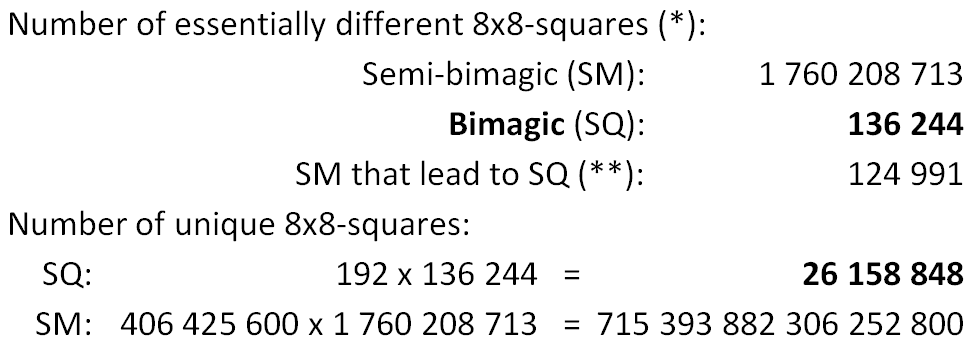All bimagic squares of order 8
 Total Number = 192 · 136 244 = 26 158 848

Abbreviations:    SQ = bimagic square of order 8    SM = semi-bimagic square of order 8
 The complete sets of all SM and SQ were found by Walter Trump and Francis Gaspalou in April 2014.
Common paper of Walter Trump and Francis Gaspalou about the calculation of all bimagic 8x8 squares (PDF)(*)  Beside rotations and reflections there are 192 permutations of rows and columns under which all magic 8x8-squares are invariant.
For SM the number of permutations is (8!/2)².
(**) By permutations of rows and columns a given SM can be transformed (or not) into one or several SQ. See PDF: SQ from same SM

Read how semi-magic 8x8-squares can be described by 16 integers.

Transformations that work on bimagic 8x8 squares.

Read what confirmations are already done and which should be done next.

Dwane Campbell could solve the hot spot problem.
There are still some open questions about the strange distribution of the squares.

- ZIP: Complete list of all essentially different SQ (9 MB)
- ZIP: List of all SM that lead to SQ (8 MB)
The second number indicates how many ess. diff. SQ can be created from a certain SM.

 Research on bimagic 8x8-squares done by Francis Gaspalou before
- PDF: How many squares are there, Mr. Tarry?
- PDF: Revisit of the method of construction of the first bimagic squares
- PDF: About the Rilly's method for generating 8x8 bimagic squares
- PDF: Derived Methods from those of Coccoz and Rilly

 What about calculating all bimagic 9x9-squares?
In 2005 I estimated the number of bimagic squares by stochastical methods (estimates of multimagic squares).
The number of bimagic 8x8-squares was estimated to 2×1007. Now we know the correct number: 2.6×1007. That is a little greater, because of weak symmetry advantages.
We expect about 1022 bimagic squares of order 9. That is so very very much more. A calculation is definitively not possible with our methods.
But it should be possible to determine certain subsets, like the centrally symmetrical (= associative) bimagic squares of order 9.

 Home: Notes on Magic Squares Walter Trump, Nürnberg, Germany, (c) 2014-05-28, last update 2017-10-03